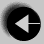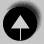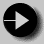## Pointers in C

Pointers are not exclusive to functions, but this seems a good place to introduce the pointer type.

Imagine that we have an int called i. Its address could be represented by the symbol &i. If the pointer is to be stored as a variable, it should be stored like this.

```
int *pi = &i;```

int * is the notation for a pointer to an int. & is the operator which returns the address of its argument. When it is used, as in &i we say it is referencing i.

The opposite operator, which gives the value at the end of the pointer is *. An example of use, known as de-referencing pi, would be

```
i = *pi;```

Take care not to confuse the many uses of the * sign; Multiplication, pointer declaration and pointer de-referencing.

This is a very confusing subject, so let us illustrate it with an example. The following function fiddle takes two arguments, x is an int while y is a pointer to int. It changes both values.

```
fiddle(int x, int *y)
{   printf(" Starting fiddle: x = %d, y = %d\n", x, *y);
x ++;
(*y)++;
printf("Finishing fiddle: x = %d, y = %d\n", x, *y);
}```

since y is a pointer, we must de-reference it before incrementing its value.

A very simple program to call this function might be as follows.

```
main()
{   int i = 0;
int j = 0;

printf(" Starting main  : i = %d, j = %d\n", x, y);
printf("Calling fiddle now\n");.
fiddle(i, &j);
printf("Returned from fiddle\n");
printf("Finishing main  : i = %d, j = %d\n", x, y);
}```

Note here how a pointer to int is created using the & operator within the call fiddle(i, &j);.

The result of running the program will look like this.

```
Starting main  : i =   0 ,j =   0
Calling fiddle now
Starting fiddle: x =   0, y =   0
Finishing fiddle: x =   1, y =   1
Returned from fiddle
Finishing main  : i =   0, j =   1```

After the return from fiddle the value of i is unchanged while j, which was passed as a pointer, has changed.

To summarise, if you wish to use arguments to modify the value of variables from a function, these arguments must be passed as pointers, and de-referenced within the function.

Where the value of an argument isn't modified, the value can be passed without any worries about pointers.Go to Modifying FunctionsGo to IndexGo to Arrays and Pointers